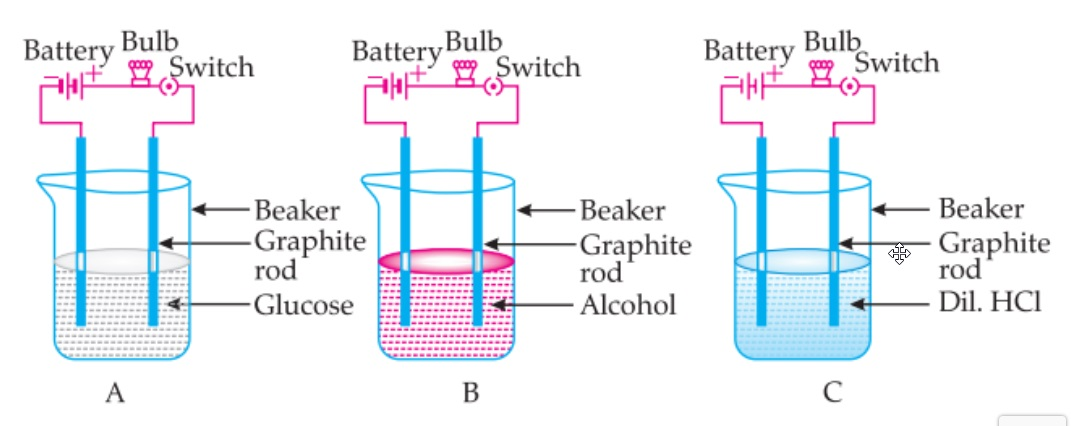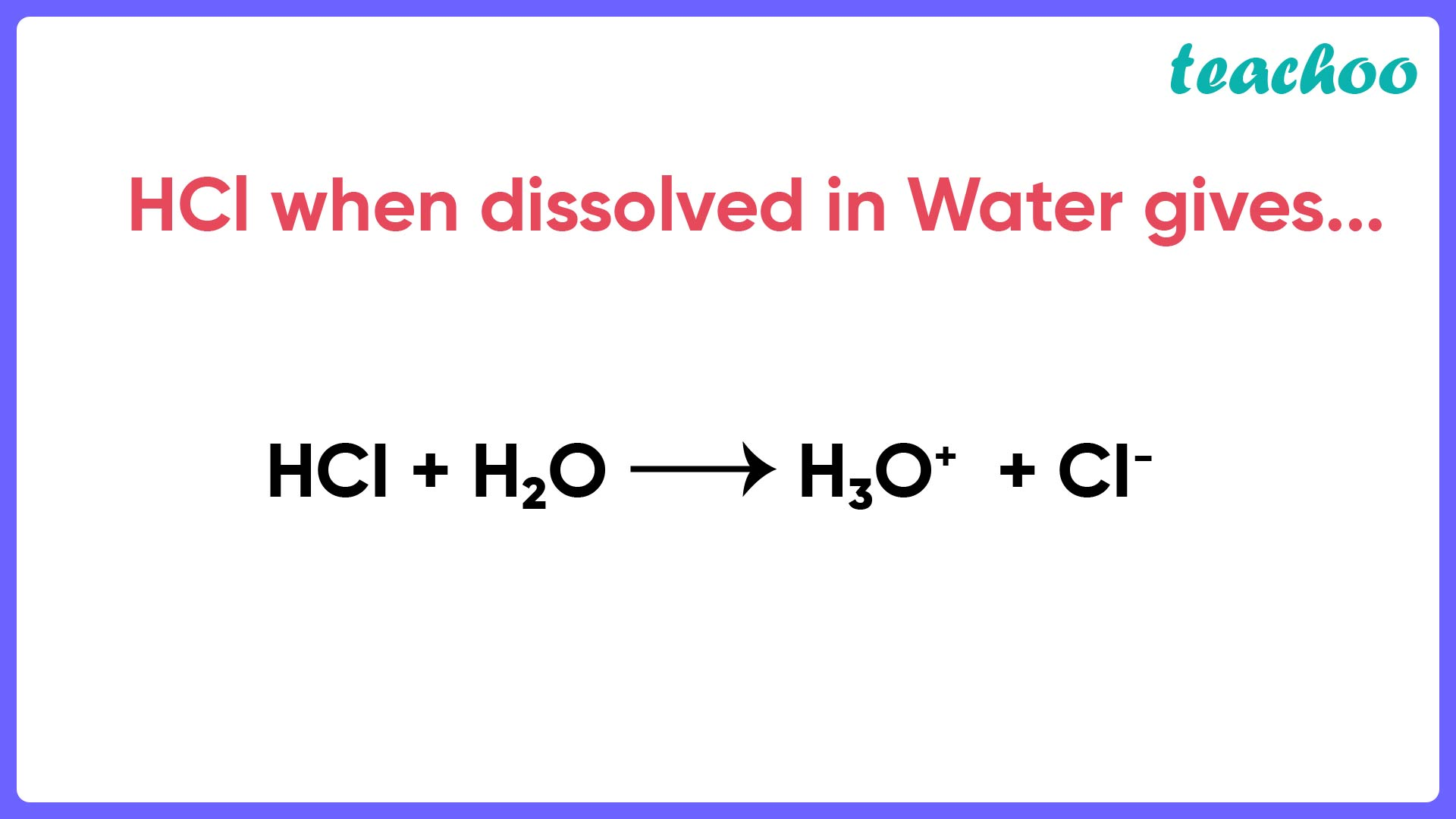Case Based Questions (MCQ)

Class 10
Chapter 2 Class 10 - Acids, Bases and Salts

## Suhana takes three beakers A, B and C filled with aqueous solutions of glucose, alcohol and hydrochloric acid  respectively as shown in the following figure:## (iv) All the bulbs glow.

Ans.

We know that Acids and bases conduct electricity in Water. They conduct electricity because they form ions in water.

Here,

• Since Glucose is neither an acid or base, it does not conduct electricity. So, it will not glow.
• Alcohol forms hydrogen bonds with water so it does not dissociate to give ions. So, the bulb will not glow .
• Both glucose and alcohol are non electrolytes i.e. they do not produce ions when dissolved in water.

Due to this, they are unable to conduct electricity and hence, the bulbs A and B do not glow.

On the other hand, dil HCl is an electrolyte i.e. it produces ions when dissolved in water. So, they are able to conduct electricity and hence, the bulb C glows.

So, the correct answer is (i) Bulb A and B do not glow but bulb C glows.

## (iv) a non electrolyte

Ans.

An electrolyte is a solution which is able to produce ions in aqueous solution.

These ions are responsible for the conduction of electricity .

Therefore, a bulb will only glow if we take an electrolyte because only then ions will be produced and the solution will be able to conduct electricity.

Note : Both Acids and Bases are electrolytes.

So, the correct answer is (ii) an electrolyte

## (iv) Unionized HCl

Ans.

Aqueous solution of HCl means HCl in water.

So, the reaction looks likeA dilute aqueous solution of hydrochloric acid is an electrolyte and produces H 3 O + and Cl - ions .

So, the correct answer is (i)

## (iv) The bulb glows in NaOH solution as well as in alcohol.

Ans.

Here,

• Alcohol forms hydrogen bonds with water so it does not dissociate to give ions. So, the bulb will not glow .
• And NaOH is a base. Since base is an electrolyte , it is able to produce ions and thereby conduct electricity.

Therefore, the bulb will glow if we replace alcohol with NaOH.

So, the correct answer is (ii) The bulb will glow in NaOH solution but not in  alcohol.

Learn in your speed, with individual attention - Teachoo Maths 1-on-1 Class